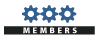# forza

Members

7

0 Neutral

## About forza• Rank
Nemam pravo na custom titulu!
1. miza ti si kidalica ...
2. miza i padash za repku
3. miza objasnjava,rank 1 plus 100 drop hackova . .
4. gg clan + ,gg srne :_) gg orgaa..
5. gl sa turom :)))
6. brate da okacim onu sliku za huga :)))))))))))))))))))))))
7. bex rdy :))))))))))))))))))